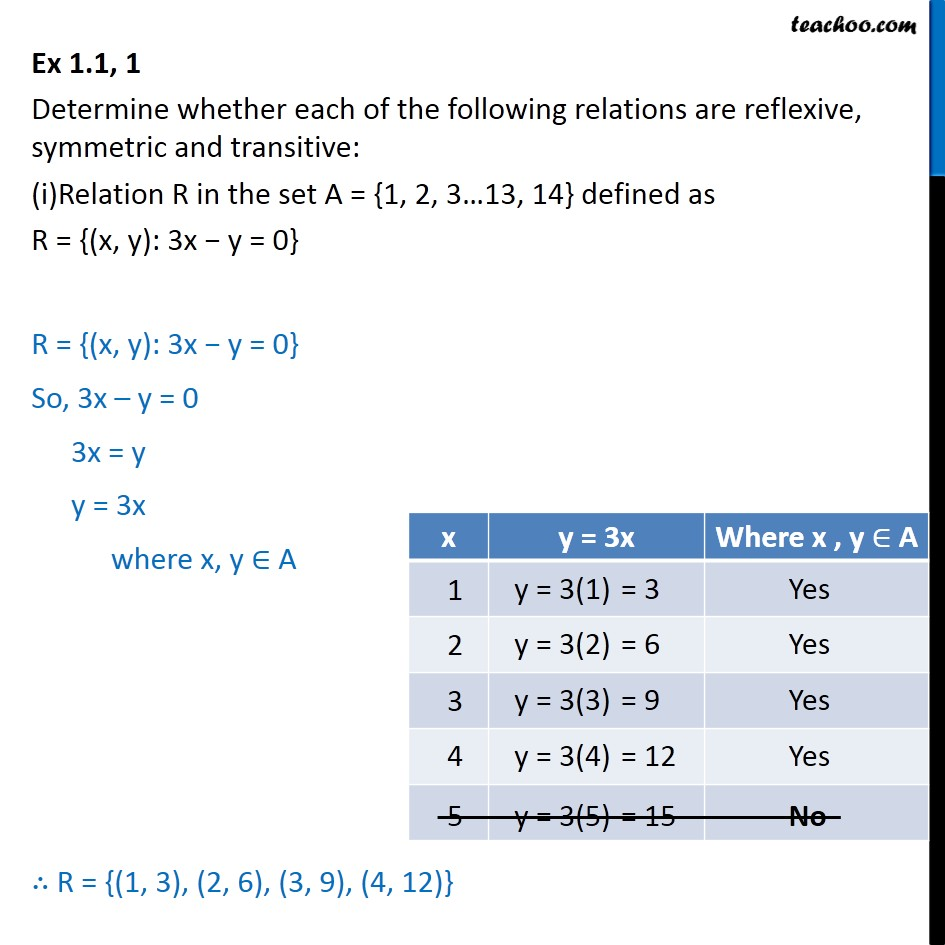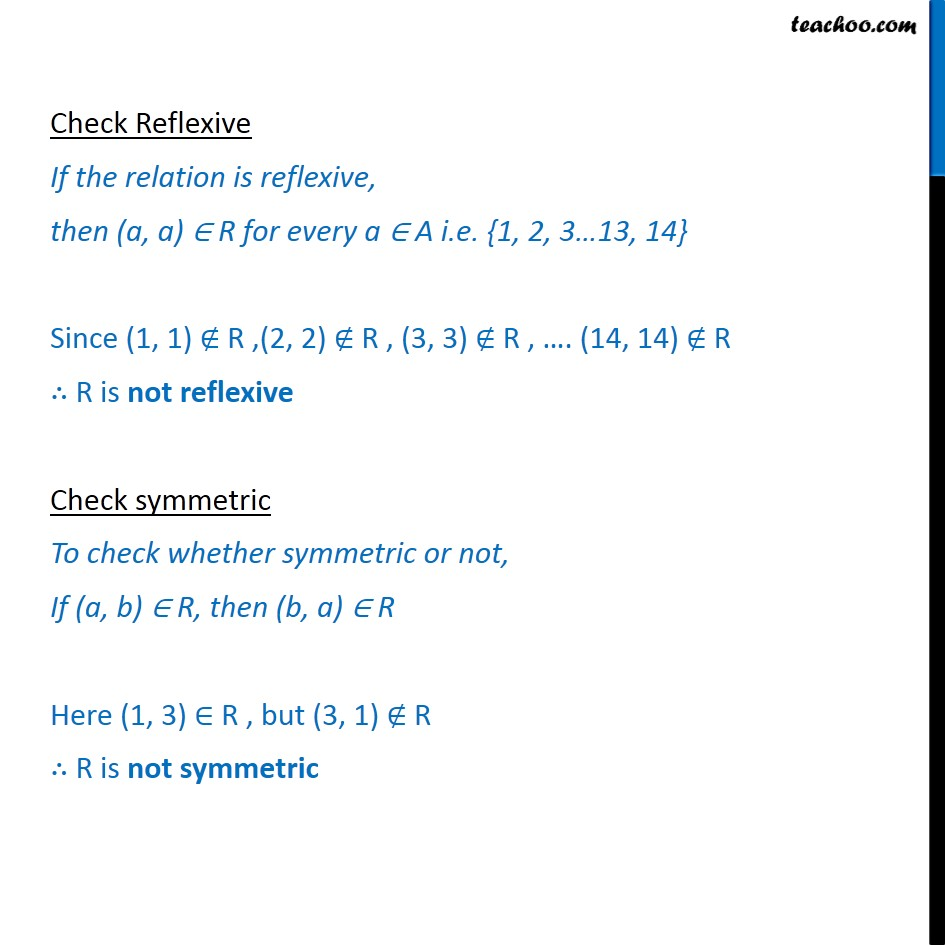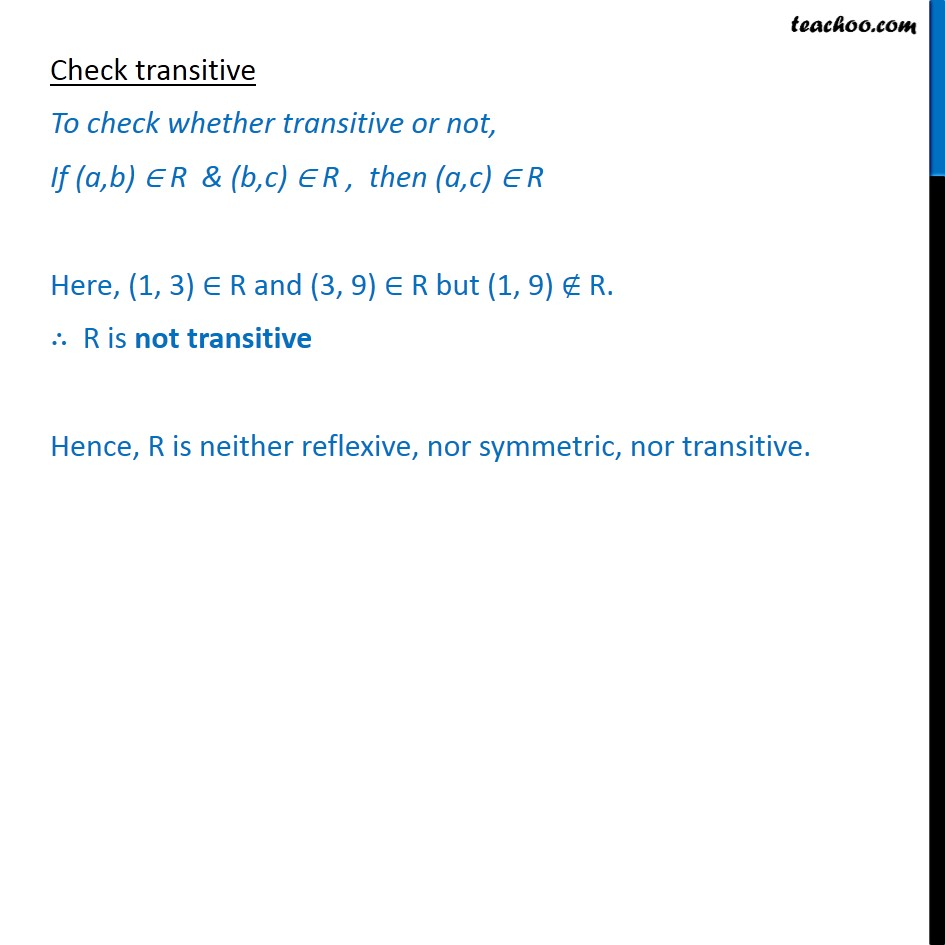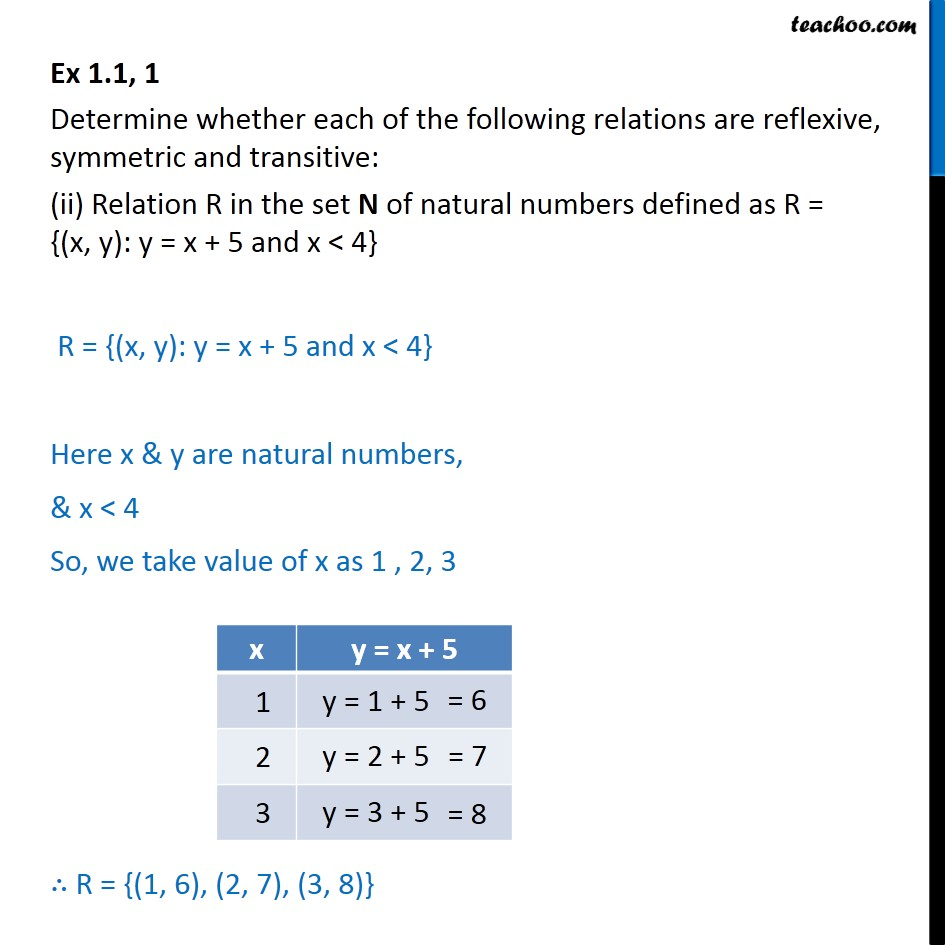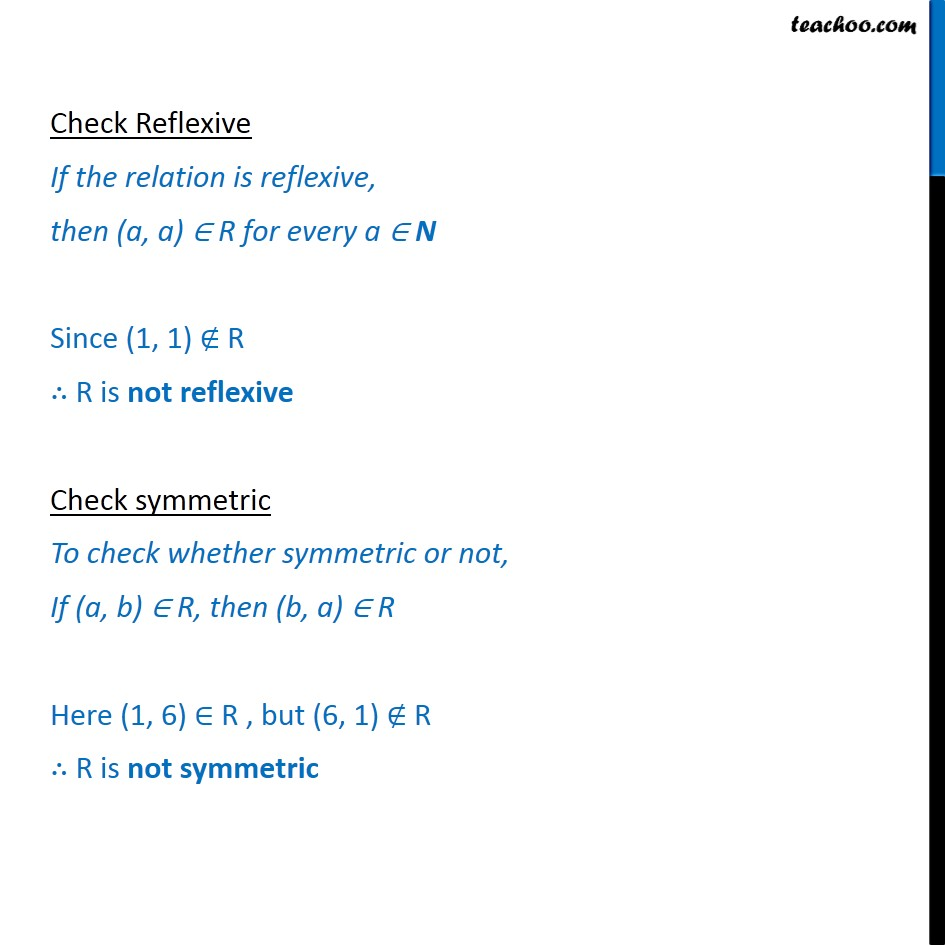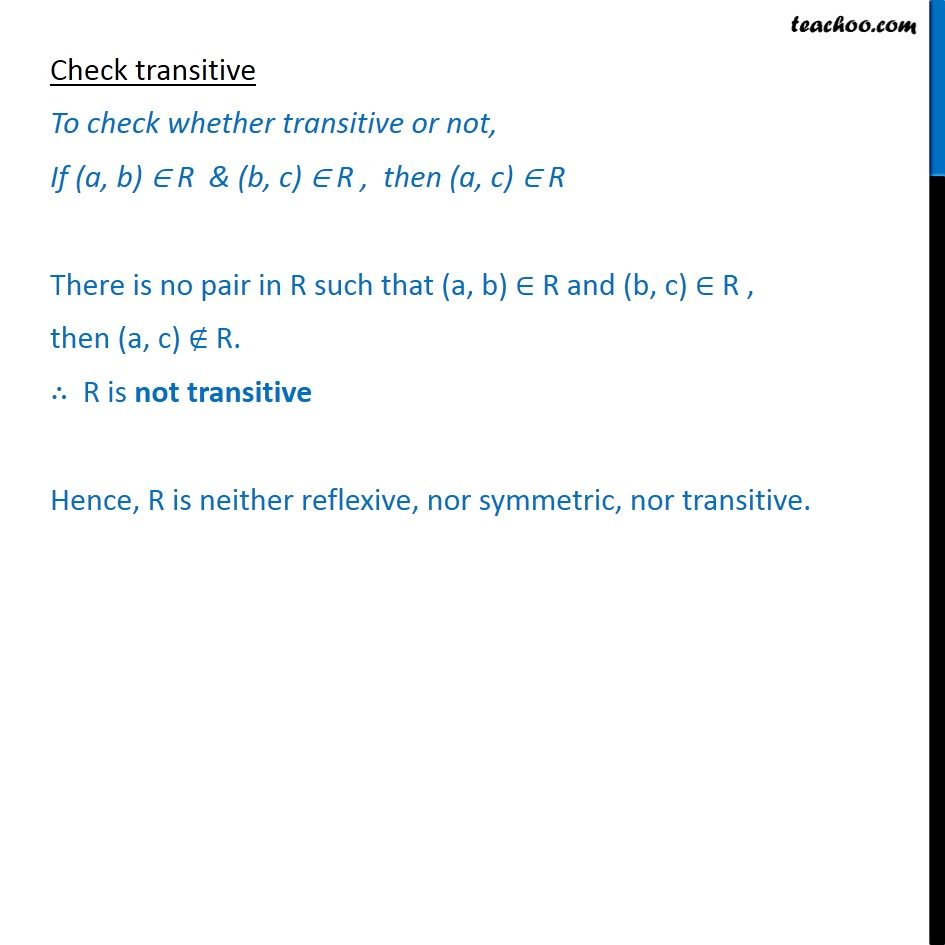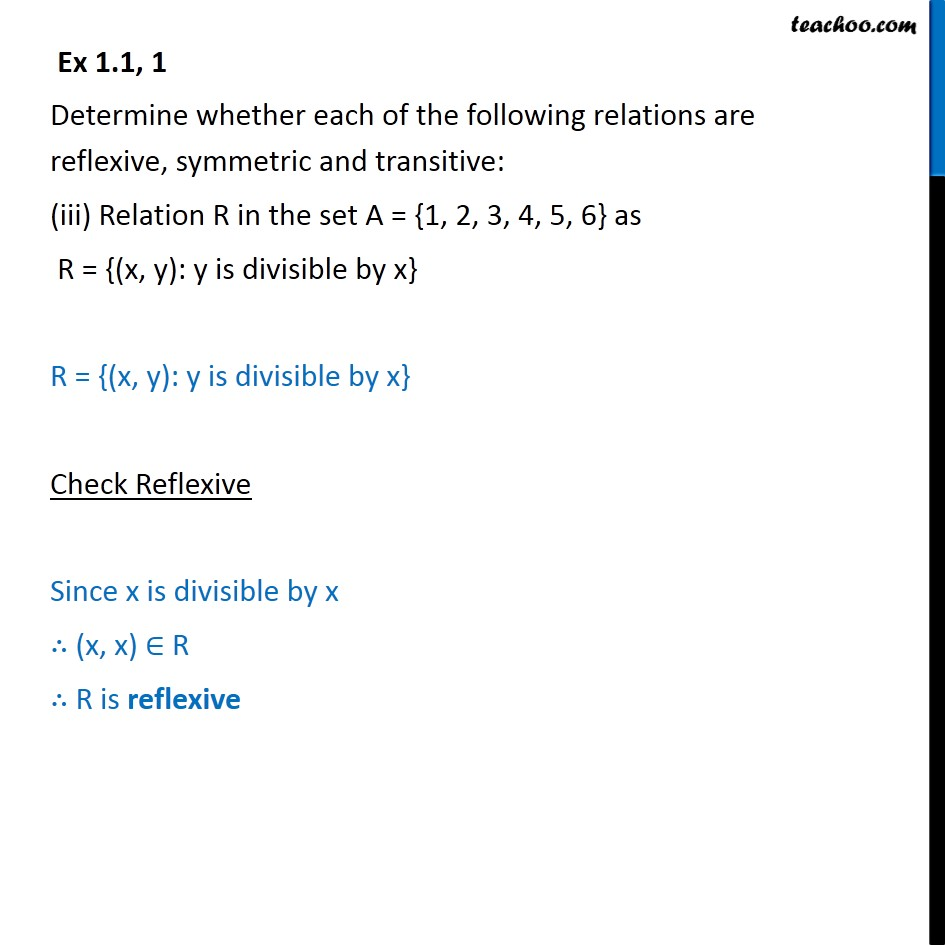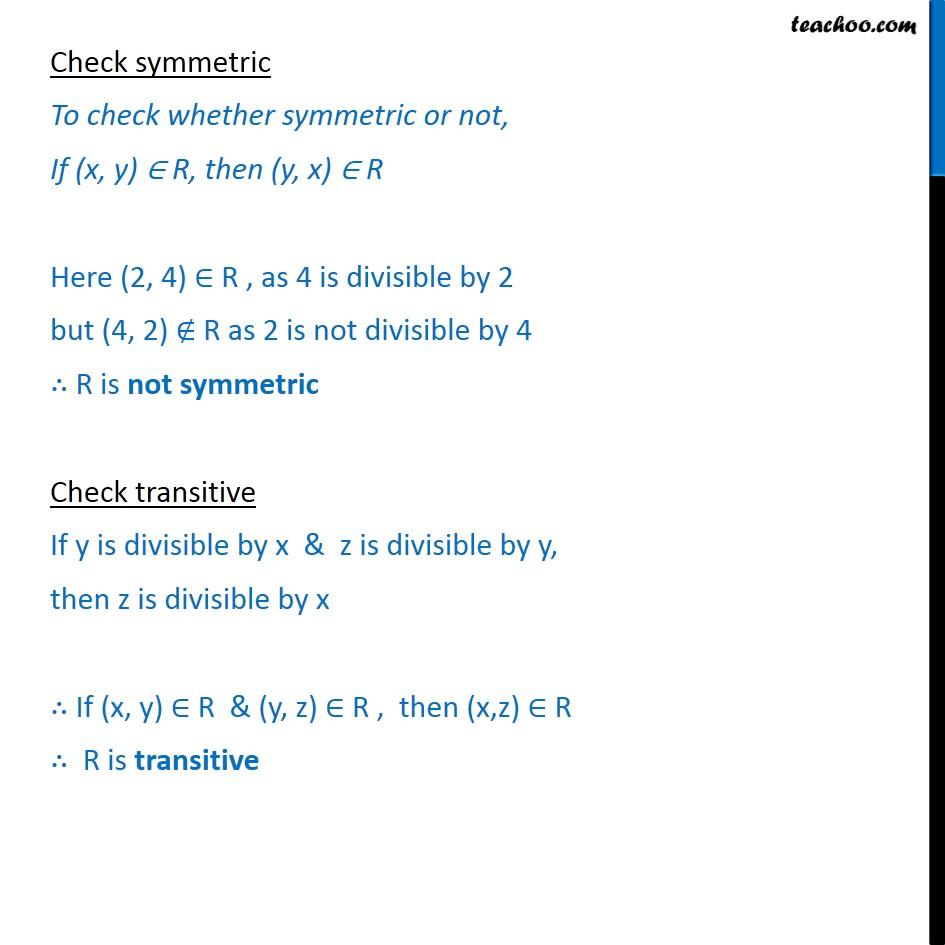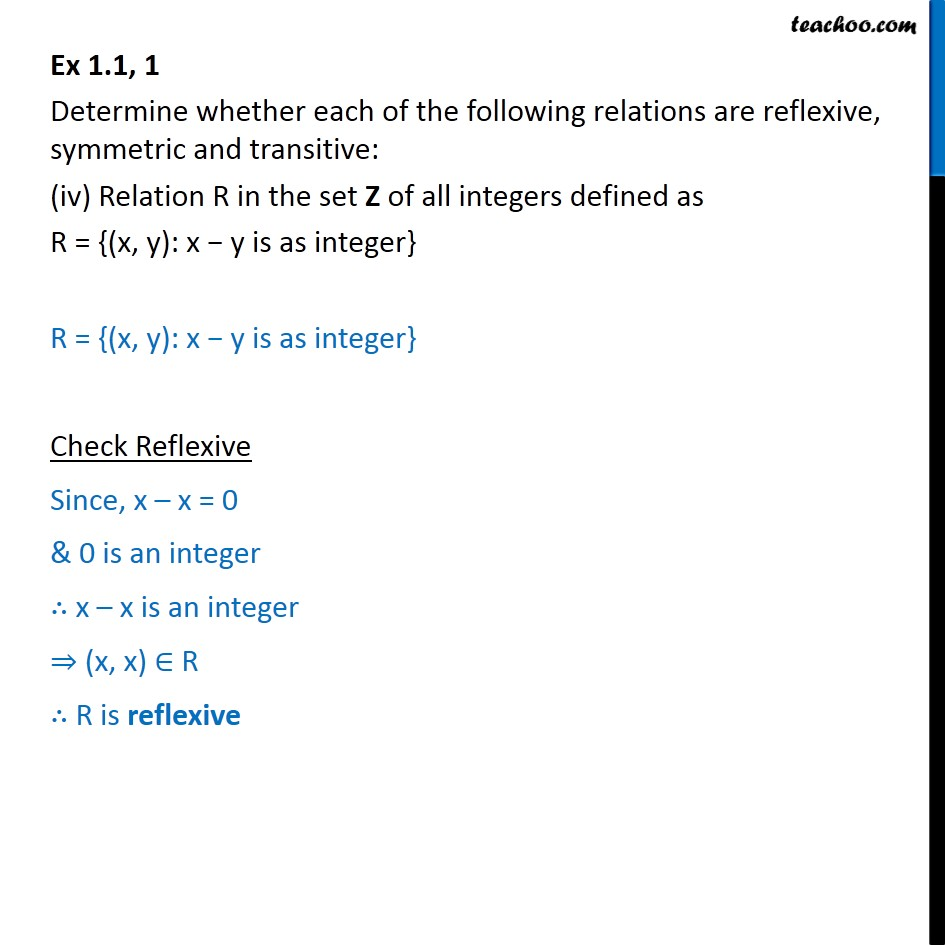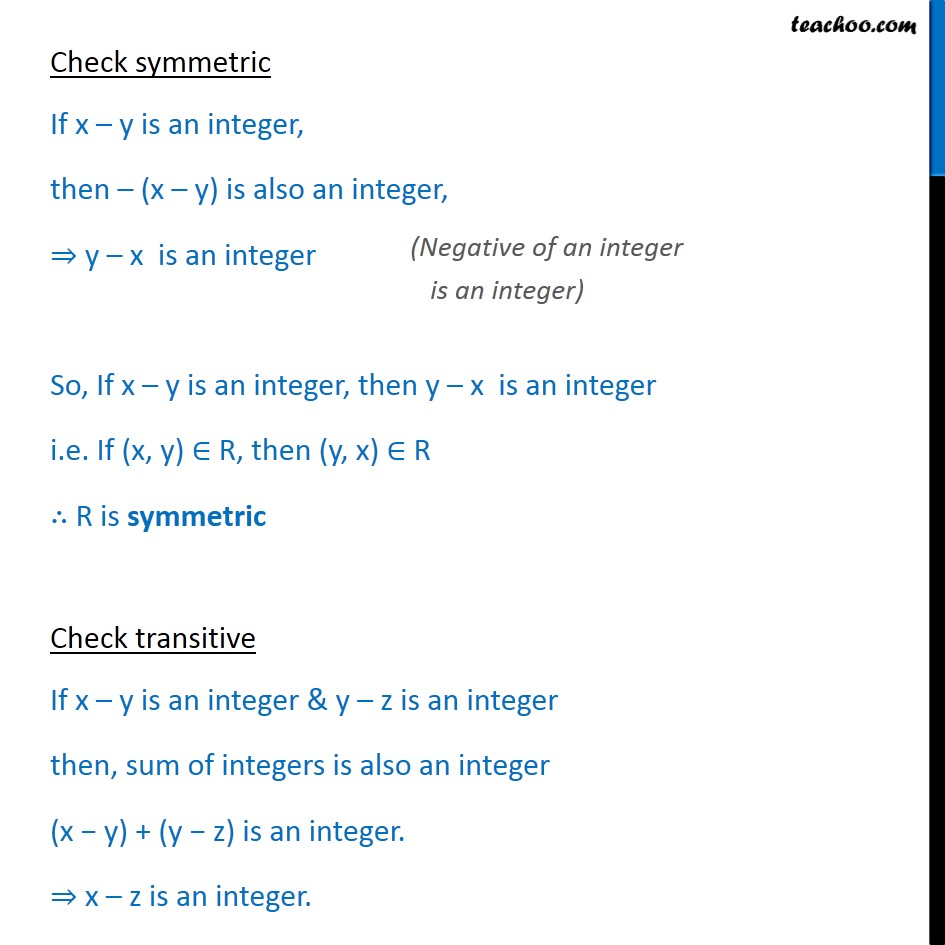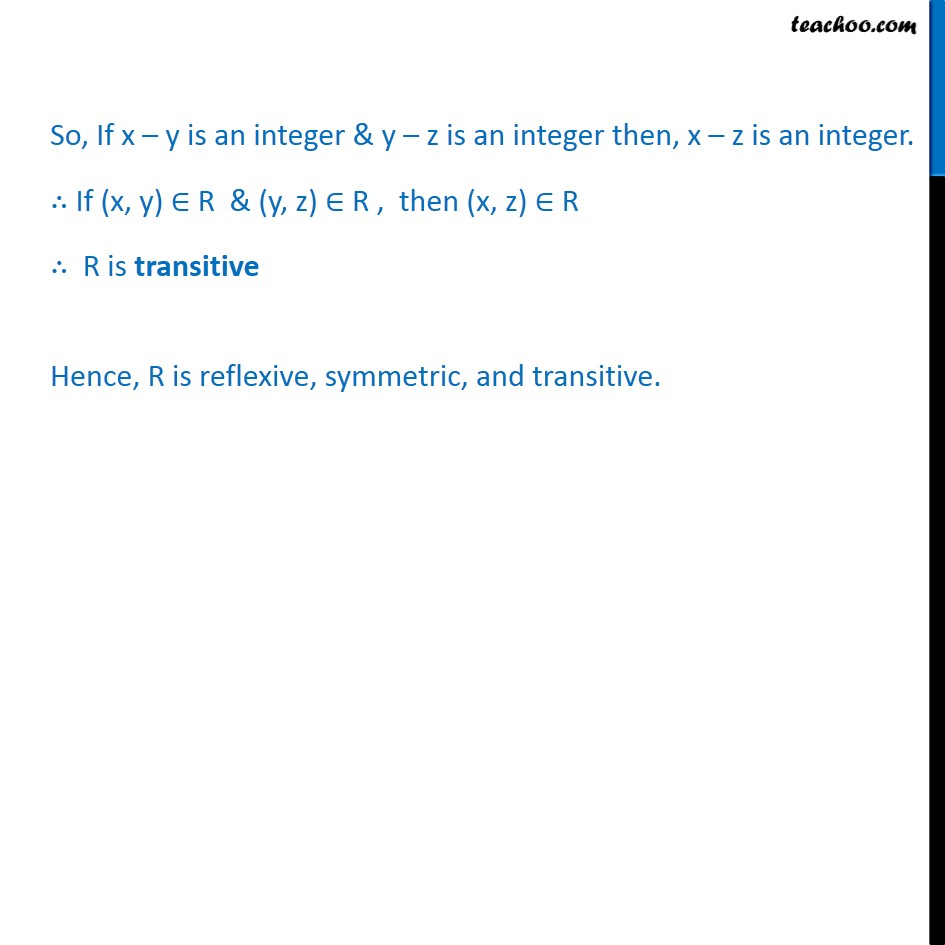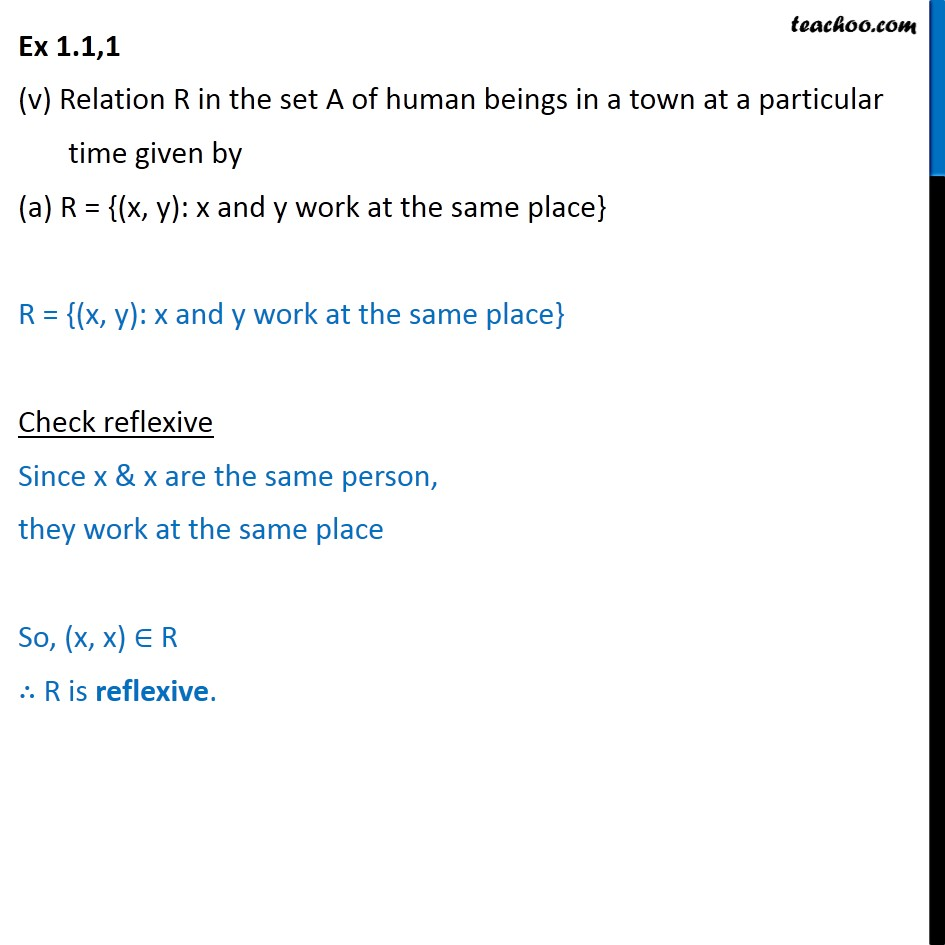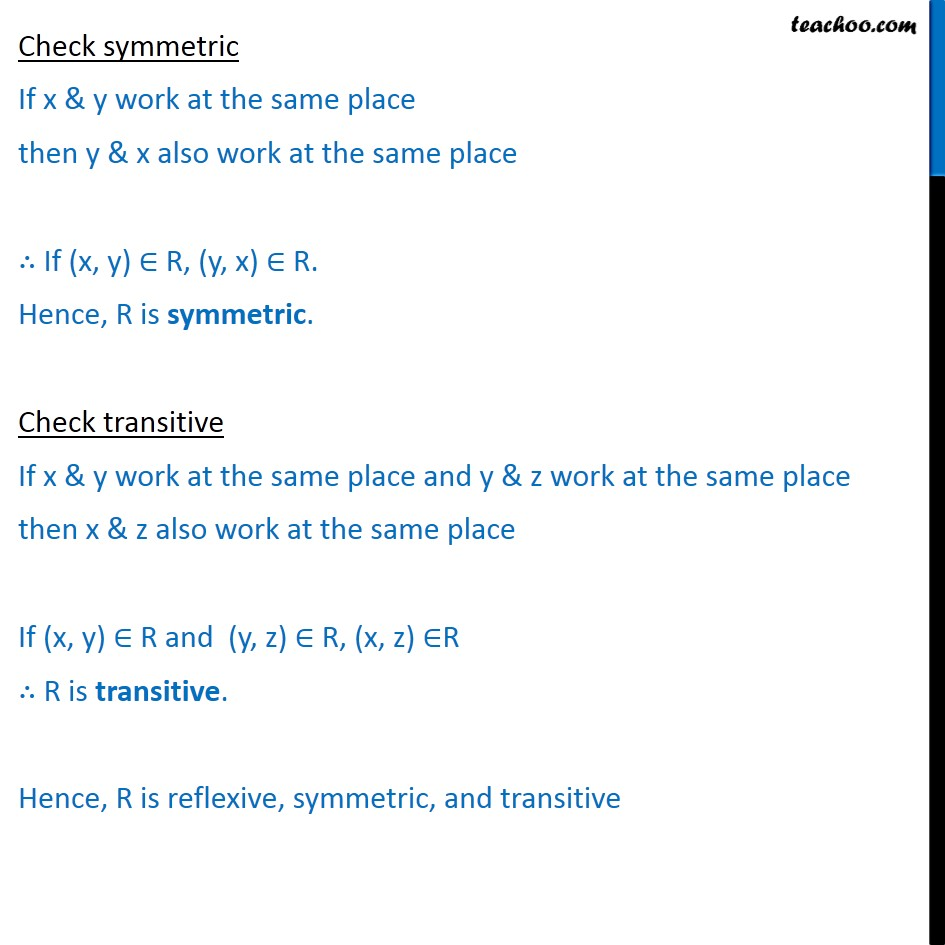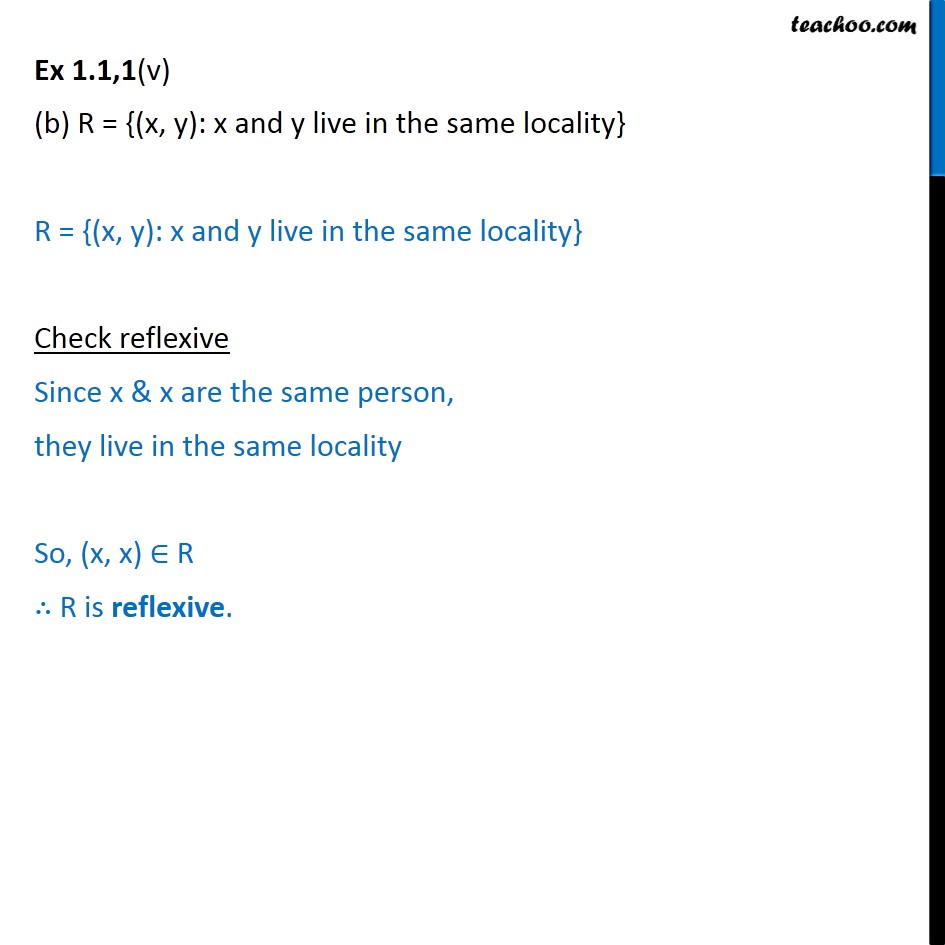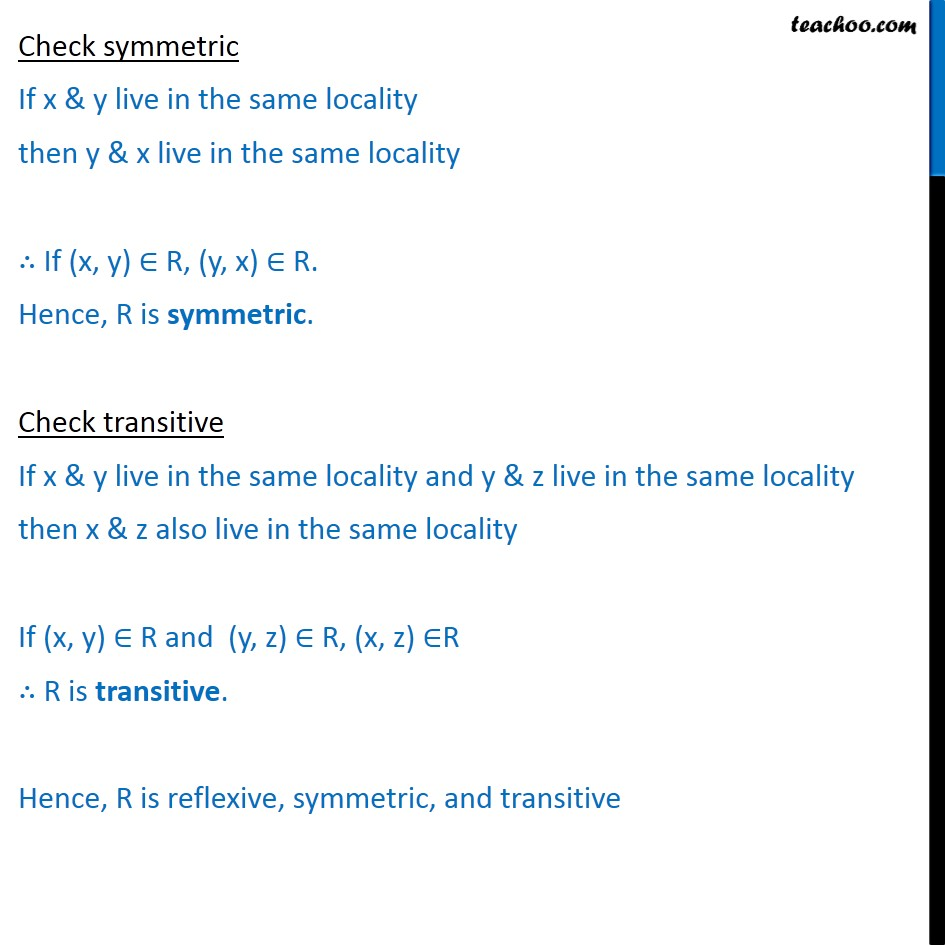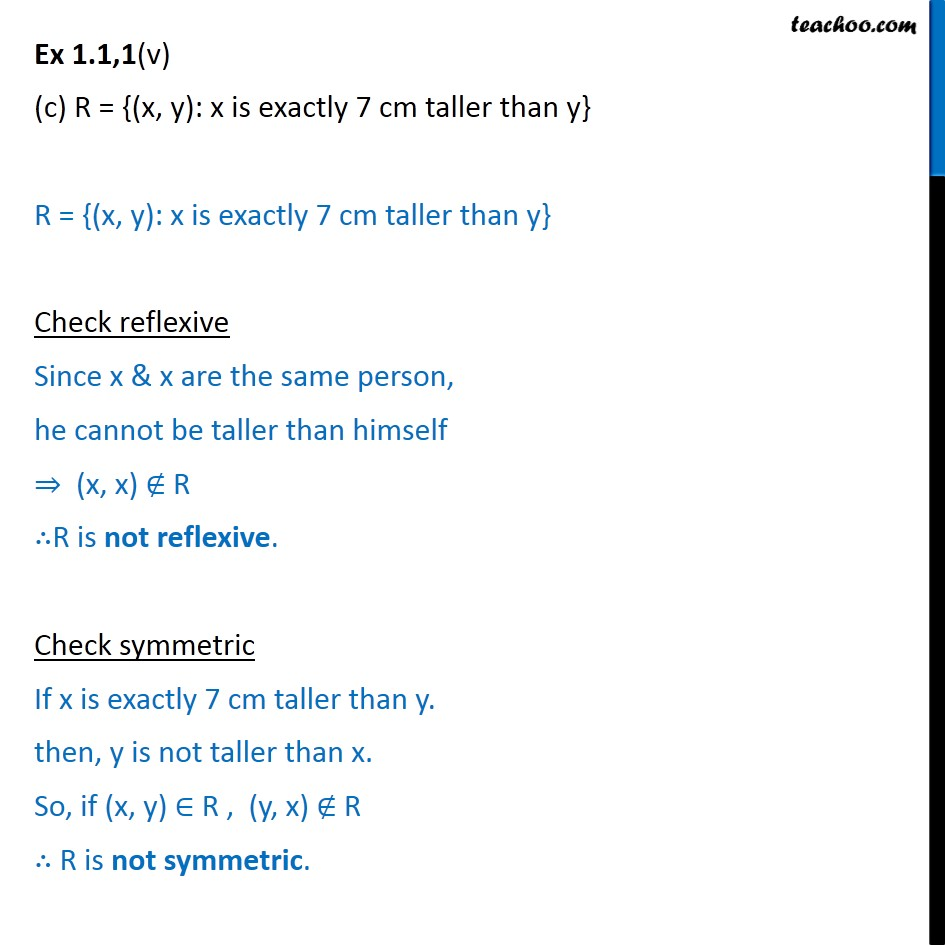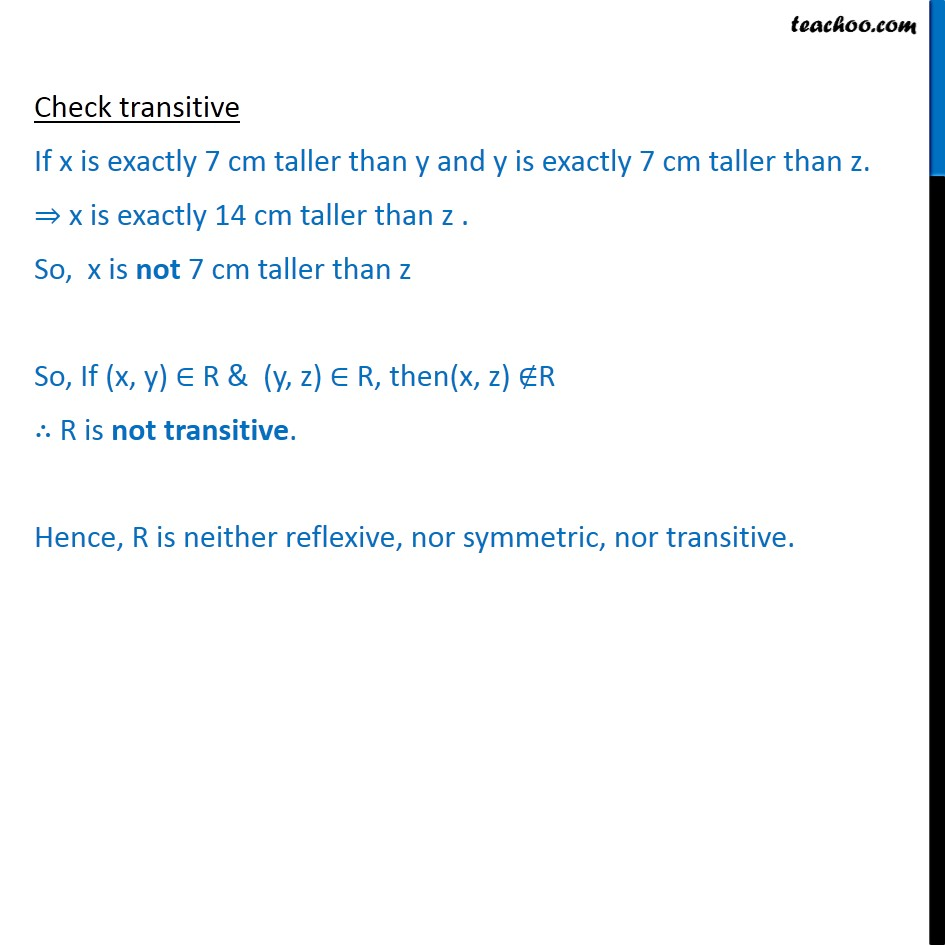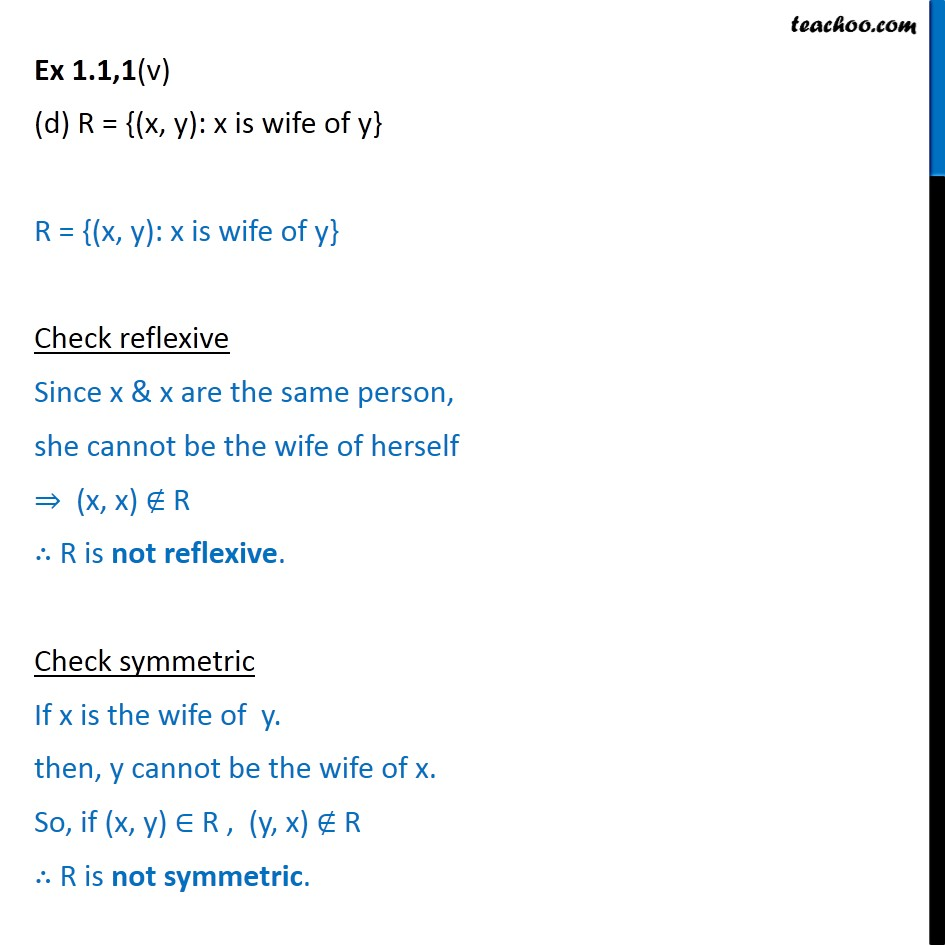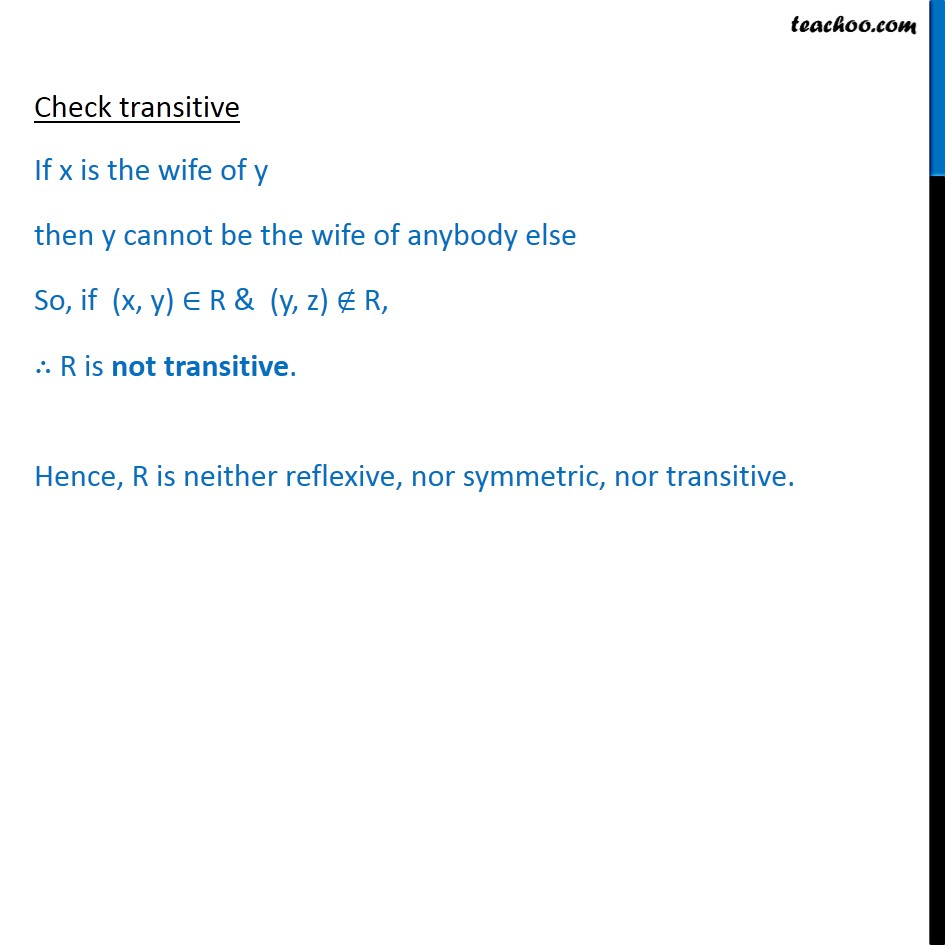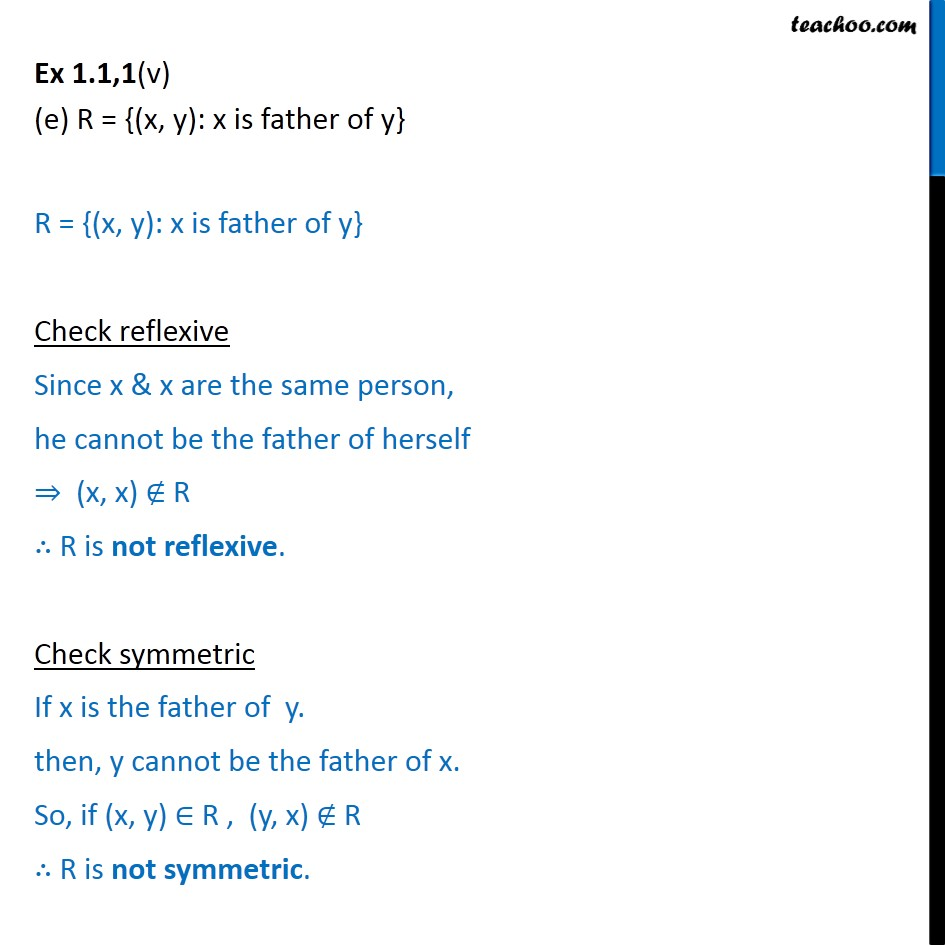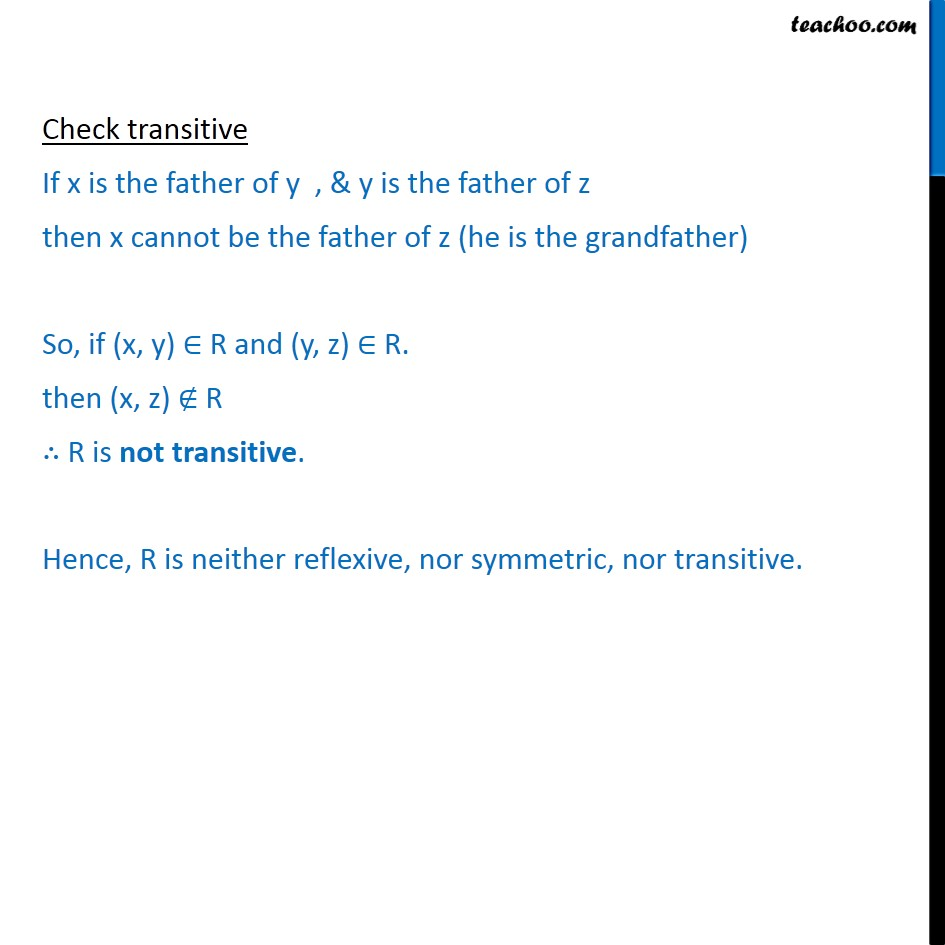Subscribe to our Youtube Channel - https://you.tube/teachoo

1. Chapter 1 Class 12 Relation and Functions
2. Serial order wise
3. Ex 1.1

Transcript

Ex 1.1, 1 Determine whether each of the following relations are reflexive, symmetric and transitive: (i)Relation R in the set A = {1, 2, 3 13, 14} defined as R = {(x, y): 3x y = 0} R = {(x, y): 3x y = 0} So, 3x y = 0 3x = y y = 3x where x, y A R = {(1, 3), (2, 6), (3, 9), (4, 12)} Check Reflexive If the relation is reflexive, then (a, a) R for every a A i.e. {1, 2, 3 13, 14} Since (1, 1) R ,(2, 2) R , (3, 3) R , . (14, 14) R R is not reflexive Check symmetric To check whether symmetric or not, If (a, b) R, then (b, a) R Here (1, 3) R , but (3, 1) R R is not symmetric Check transitive To check whether transitive or not, If (a,b) R & (b,c) R , then (a,c) R Here, (1, 3) R and (3, 9) R but (1, 9) R. R is not transitive Hence, R is neither reflexive, nor symmetric, nor transitive. Ex 1.1, 1 Determine whether each of the following relations are reflexive, symmetric and transitive: (ii) Relation R in the set N of natural numbers defined as R = {(x, y): y = x + 5 and x < 4} R = {(x, y): y = x + 5 and x < 4} Here x & y are natural numbers, & x < 4 So, we take value of x as 1 , 2, 3 R = {(1, 6), (2, 7), (3, 8)} Check Reflexive If the relation is reflexive, then (a, a) R for every a N Since (1, 1) R R is not reflexive Check symmetric To check whether symmetric or not, If (a, b) R, then (b, a) R Here (1, 6) R , but (6, 1) R R is not symmetric Check transitive To check whether transitive or not, If (a, b) R & (b, c) R , then (a, c) R There is no pair in R such that (a, b) R and (b, c) R , then (a, c) R. R is not transitive Hence, R is neither reflexive, nor symmetric, nor transitive. Ex 1.1, 1 Determine whether each of the following relations are reflexive, symmetric and transitive: (iii) Relation R in the set A = {1, 2, 3, 4, 5, 6} as R = {(x, y): y is divisible by x} R = {(x, y): y is divisible by x} Check Reflexive Since x is divisible by x (x, x) R R is reflexive Check symmetric To check whether symmetric or not, If (x, y) R, then (y, x) R Here (2, 4) R , as 4 is divisible by 2 but (4, 2) R as 2 is not divisible by 4 R is not symmetric Check transitive If y is divisible by x & z is divisible by y, then z is divisible by x If (x, y) R & (y, z) R , then (x,z) R R is transitive Ex 1.1, 1 Determine whether each of the following relations are reflexive, symmetric and transitive: (iv) Relation R in the set Z of all integers defined as R = {(x, y): x y is as integer} R = {(x, y): x y is as integer} Check Reflexive Since, x x = 0 & 0 is an integer x x is an integer (x, x) R R is reflexive Check symmetric If x y is an integer, then (x y) is also an integer, y x is an integer So, If x y is an integer, then y x is an integer i.e. If (x, y) R, then (y, x) R R is symmetric Check transitive If x y is an integer & y z is an integer then, sum of integers is also an integer (x y) + (y z) is an integer. x z is an integer. So, If x y is an integer & y z is an integer then, x z is an integer. If (x, y) R & (y, z) R , then (x, z) R R is transitive Hence, R is reflexive, symmetric, and transitive. Ex 1.1,1 (v) Relation R in the set A of human beings in a town at a particular time given by (a) R = {(x, y): x and y work at the same place} R = {(x, y): x and y work at the same place} Check reflexive Since x & x are the same person, they work at the same place So, (x, x) R R is reflexive. Check symmetric If x & y work at the same place then y & x also work at the same place If (x, y) R, (y, x) R. Hence, R is symmetric. Check transitive If x & y work at the same place and y & z work at the same place then x & z also work at the same place If (x, y) R and (y, z) R, (x, z) R R is transitive. Hence, R is reflexive, symmetric, and transitive Ex 1.1,1(v) (b) R = {(x, y): x and y live in the same locality} R = {(x, y): x and y live in the same locality} Check reflexive Since x & x are the same person, they live in the same locality So, (x, x) R R is reflexive. Check symmetric If x & y live in the same locality then y & x live in the same locality If (x, y) R, (y, x) R. Hence, R is symmetric. Check transitive If x & y live in the same locality and y & z live in the same locality then x & z also live in the same locality If (x, y) R and (y, z) R, (x, z) R R is transitive. Hence, R is reflexive, symmetric, and transitive Ex 1.1,1(v) (c) R = {(x, y): x is exactly 7 cm taller than y} R = {(x, y): x is exactly 7 cm taller than y} Check reflexive Since x & x are the same person, he cannot be taller than himself (x, x) R R is not reflexive. Check symmetric If x is exactly 7 cm taller than y. then, y is not taller than x. So, if (x, y) R , (y, x) R R is not symmetric. Check transitive If x is exactly 7 cm taller than y and y is exactly 7 cm taller than z. x is exactly 14 cm taller than z . So, x is not 7 cm taller than z So, If (x, y) R & (y, z) R, then(x, z) R R is not transitive. Hence, R is neither reflexive, nor symmetric, nor transitive. Ex 1.1,1(v) (d) R = {(x, y): x is wife of y} R = {(x, y): x is wife of y} Check reflexive Since x & x are the same person, she cannot be the wife of herself (x, x) R R is not reflexive. Check symmetric If x is the wife of y. then, y cannot be the wife of x. So, if (x, y) R , (y, x) R R is not symmetric. Check transitive If x is the wife of y then y cannot be the wife of anybody else So, if (x, y) R & (y, z) R, R is not transitive. Hence, R is neither reflexive, nor symmetric, nor transitive. Ex 1.1,1(v) (e) R = {(x, y): x is father of y} R = {(x, y): x is father of y} Check reflexive Since x & x are the same person, he cannot be the father of herself (x, x) R R is not reflexive. Check symmetric If x is the father of y. then, y cannot be the father of x. So, if (x, y) R , (y, x) R R is not symmetric. Check transitive If x is the father of y , & y is the father of z then x cannot be the father of z (he is the grandfather) So, if (x, y) R and (y, z) R. then (x, z) R R is not transitive. Hence, R is neither reflexive, nor symmetric, nor transitive.

Ex 1.1